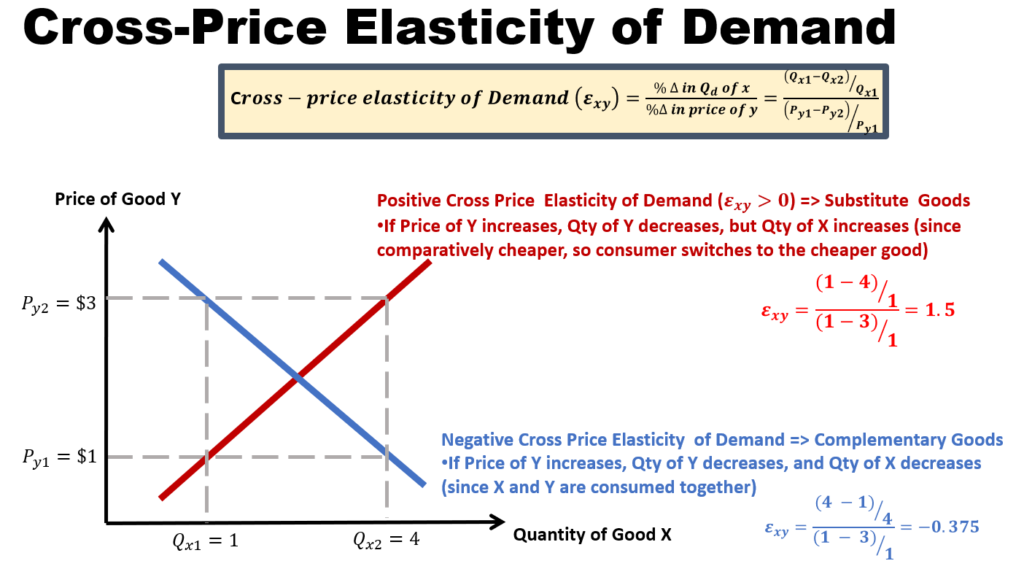# Cross-Price Elasticity of Demand

## Cross-price elasticity of demand - changes in quantity consumed of one good over changes in price of another good

Cross elasticity of Demand (Exy) refers to the degree of responsiveness of demand of good X due to a change in the price of good Y. The formula for Exy is as shown in the diagram. The sign of Exy matters (unlike the case for price elasticity of demand).

• If Exy is greater than 0, then it means that X and Y are substitutes.
• If Exy is less than 0, then it means that X and Y are complements## Substitute Goods

• Substitutes are goods which satisfy the same want and may be used in place of one another.  For example: butter and margarine.
• Substitutes have positive cross elasticity, i.e., cross elasticity is greater than 0.  Why?
• Butter and margarine are examples of substitutes are they fulfill the same need. If the price of butter increases, this will make it more expensive. Consumer will switch to margarine, the cheaper alternative. Hence, the demand for margarine will increase.
• As we can see, if goods X and Y are substitutes, and if price of good X increase, the quantity demanded of good Y will increase.  Using the formula Exy, the sign of the numerator and denominator cancels out, giving it a positive sign. Hence, Exy >0 will imply substitute goods.

## Complementary Goods

• Complementary goods are goods that are used together, such as cars and petrol.
• Complementary goods have negative cross elasticity, i.e., elasticity is less than 0. Why?
• Petrol and cars are examples of complementary goods. These goods need to be consumed together. if the prices of petrol increase, usage of cars become more expensive. Commuters may switch from cars to cheaper modes of transportation. Hence the quantity of cars is reduced if price of petrol increase.
• In summary, if goods X and Y are complements, and if price of good X increase, the quantity of good Y will decrease. Using the formula Exy, the sign of the numerator is different from that of the denominator, giving it a negative sign. Hence, Exy <0 will imply complementary goods.